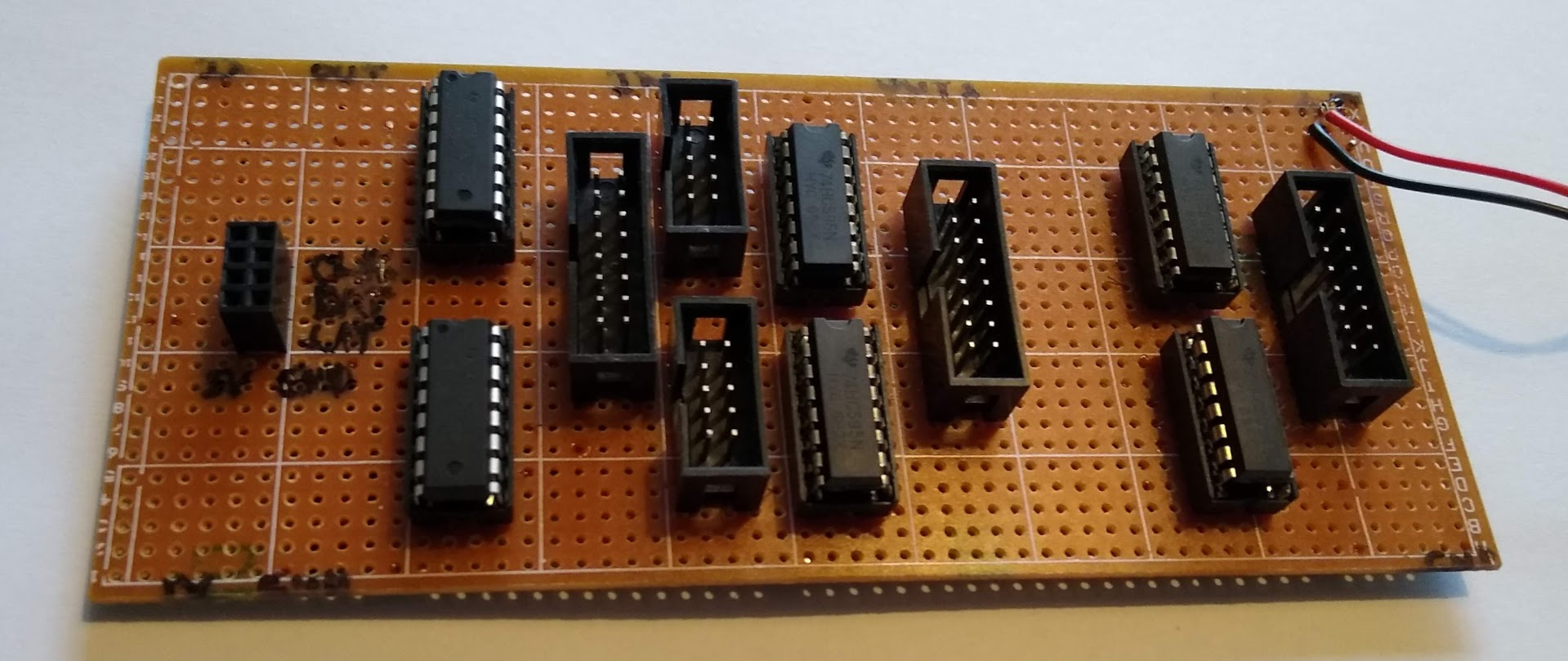# Posts

### Doom Emacs

Emacs is my preferred tool for development, statistical computing, and writing. The killer feature is without doubt https://orgmode.org/ which allows for powerful literate programming, handling of bibliographies, organizing notes collections, and much more.

I finally decided to make the move from my vanilla emacs configuration to Doom Emacs. This gives an optimized emacs experience with fast load times due to lazy-loading of packages, and more importantly, the maintainer is doing an amazing job on adapting to new features and changes to emacs and the various lisp packages. A task which I found increasingly time-consuming.

### Nonlinear latent variable models

ML-inference in non-linear SEMs is complex. Computational intensive methods based on numerical integration are needed and results are sensitive to distributional assumptions.

In a recent paper: A two-stage estimation procedure for non-linear structural equation models by Klaus Kähler Holst & Esben Budtz-Jørgensen (https://doi.org/10.1093/biostatistics/kxy082), we consider two-stage estimators as a computationally simple alternative to MLE. Here both steps are based on linear models: first we predict the non-linear terms and then these are related to latent outcomes in the second step.

### Logic gates

Logic gates are the building blocks of digital electronics. Simple logic gates are efficiently implemented in various IC packages such as the 74HCXX series. However, it is educational to have a look at the implementation using just NPN transistors.

### Shift register circuit board

The 74HC165 and 74HC495 are useful integrated circuits for dealing with multiple digital inputs and outputs. In a recent project, I used the following prototype based on a variant of the above simple circuit schema:The four 74HC495 ICs gives access to 2 x 16 bits accessible through IDC connectors controllable using just three pins on the microcontroller (DATA, CLOCK, LATCH). Similarly, two 74HC165 ICs gives access to 16 input bits through another IDC connector.

### A simple ODE Class

A small illustration on using the armadillo C++ linear algebra library for solving an ordinary differential equation of the form $X’(t) = F(t,X(t),U(t)).$

The abstract super class Solver defines the methods solve (for approximating the solution in user-defined time-points) and solveint (for interpolating user-defined input functions on finer grid). As an illustration a simple Runge-Kutta solver is derived in the class RK4.

The first step is to define the ODE, here a simple one-dimensional ODE $$X’(t) = \theta\cdot\{U(t)-X(t)\}$$ with a single input $$U(t)$$:

rowvec dX(const rowvec &input, // time (first element) and additional input variables
const rowvec &x,     // state variables
const rowvec &theta) {   // parameters
rowvec res = { theta(0)*theta(1)*(input(1)-x(0)) };
return( res );
}


The ODE may then be solved using the following syntax

odesolver::RK4 MyODE(dX);
arma::mat res = MyODE.solve(input, init, theta);


with the step size defined implicitly by input (first column is the time variable and the following columns the optional different input variables) and boundary conditions defined by init.

### Shift register (output)

The 74HC595: an 8-bit serial-in/serial or parallel-out shift register with a storage register and 3-state outputs.

If higher load is required there is also the TPIC6C595 (e.g., for driving LEDs), or it should be paired with for example ULN2803 or similar. For multiple inputs see the 74HC165.

$\newcommand{\pr}{\mathbb{P}}\newcommand{\E}{\mathbb{E}}$ Relative risks (and risk differences) are collapsible and generally considered easier to interpret than odds-ratios. In a recent publication Richardson et al (JASA, 2017) proposed a new regression model for a binary exposure which solves the computational problems that are associated with using for example binomial regression with a log-link function (or identify link for the risk difference) to obtain such parameter estimates.
Let $$Y$$ be the binary response, $$A$$ binary exposure, and $$V$$ a vector of covariates, then the target parameter is
Let $$p_a(V) = \pr(Y \mid A=a, V), a\in\{0,1\}$$, the idea is then to posit a linear model for $\theta(v) = \log \big(RR(v)\big)$ and a nuisance model for the odds-product $\phi(v) = \log\left(\frac{p_{0}(v)p_{1}(v)}{(1-p_{0}(v))(1-p_{1}(v))}\right)$ noting that these two parameters are variation independent which can be from the below L’Abbé plot. Similarly, a model can be constructed for the risk-difference on the following scale $\theta(v) = \mathrm{arctanh} \big(RD(v)\big).$